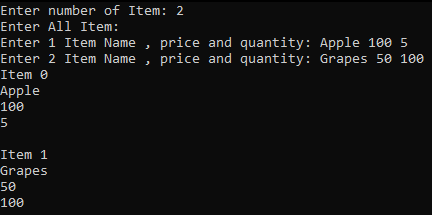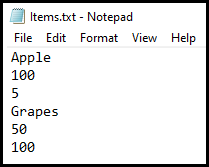# Vector Class Realtime Example in C++

## Vector Class Realtime Example in C++:

Now, we will see Vector Class Realtime Example in C++. Please read our previous article where we discussed Vector in C++ with Examples. We will write a program to store and retrieve the list of items in a file using a vector class in C++.

##### Vector Class in C++:

It is nothing but the array only. But this is not fixed sized array. This can grow and reduce by itself. How an array can reduce by itself? We have shown this in our previous articles that we have to create a bigger or small size array and transfer the elements into the new array. So, the vector automatically manages that part. This will dynamically manage the size of the array. So, this is a self-managed array. Functions available in Vector are as follows:

1. push_back(): It will insert the element at the back in an array.
2. pop_back(): It will delete the last element in the array.
3. insert(): It will insert the given value at the given index.
4. remove(): It will remove the element by the given index.
5. size(): It will return the size of the array.
6. empty(): It will check whether the array is empty or not.
##### Vector Class Realtime Example in C++:
```#include<iostream>
#include<fstream>
#include<vector>
using namespace std;

class Item
{
private:
string name;
float price;
int qty;
public:
Item ()
{
}
Item (string n, float p, int q);
friend ifstream & operator >> (ifstream & fis, Item & i);
friend ofstream & operator << (ofstream & fos, Item & i);
friend ostream & operator << (ostream & os, Item & i);
};

int main()
{
int n;
string name;
float price;
int qty;

cout << "Enter number of Item: ";
cin >> n;

vector < Item * >list;
cout << "Enter All Item: " << endl;
for (int i = 0; i < n; i++)
{
cout << "Enter " << i + 1 << " Item Name , price and quantity: ";
cin >> name;
cin >> price;
cin >> qty;
list.push_back (new Item (name, price, qty));
}

ofstream fos ("Items.txt");
vector < Item * >::iterator itr;
for (itr = list.begin (); itr != list.end (); itr++)
{
fos << **itr;
}

Item item;
ifstream fis ("Items.txt");
for (int i = 0; i < n; i++)
{
fis >> item;
cout << "Item " << i << endl << item << endl;
}
}

Item::Item (string n, float p, int q)
{
name = n;
price = p;
qty = q;
}

ofstream & operator << (ofstream & fos, Item & i)
{
fos << i.name << endl << i.price << endl << i.qty << endl;
return fos;
}

ifstream & operator >> (ifstream & fis, Item & i)
{
fis >> i.name >> i.price >> i.qty;
return fis;
}

ostream & operator << (ostream & os, Item & i)
{
os << i.name << endl << i.price << endl << i.qty << endl;
return os;
}
```
###### Output:In the next article, I am going to discuss List Class Functions in C++ with Examples. Here, in this article, we discussed Vector Class Realtime Example in C++ and I hope you enjoy this article.